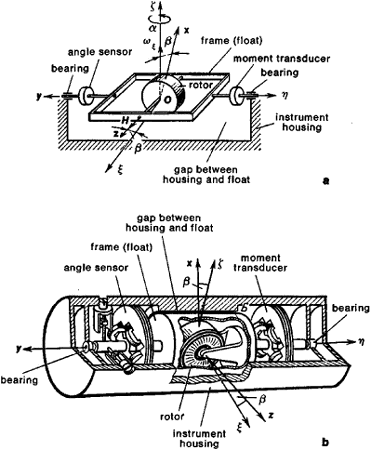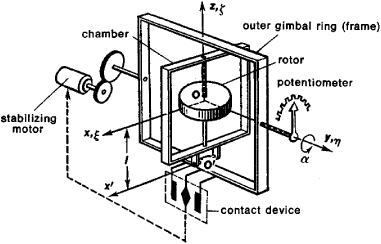# Gyroscopic Integrator

The following article is from The Great Soviet Encyclopedia (1979). It might be outdated or ideologically biased.

## Gyroscopic Integrator

a gyroscopic device containing a so-called integrating gyroscope, which is used to determine the integral of the quantity acting on the device. A distinction is made between gyroscopic integrators of angular velocity and linear accelerations.

Angular-velocity gyroscopic integrators are used to determine the angle of rotation of an object. The most advanced gyroscopic integrator is the flotation integrator (Figure 1). The rotor of the gyroscope is mounted in the frame, which consists of a cylindrical float; the axis of rotation Oy () is mounted on bearings, which are located within the body of the instrument, which is also cylindrical. The gap between the float and the body, as well as the entire free space within the body, is filled with a high-density fluid. The above system forms a fluid suspension. The buoyant force of the fluid must be equal to the weight of the gyroscopic assembly. In this case, the suspension bearings are virtually load-free. The fluid in the gap between the cylindrical surfaces of the float and the body of the instrument provides damping, whose moment is proportional to the angular speed of rotation of the float. The use of a fluid suspension partially protects the suspension axle (the axis of rotation of the float) from the action of vibrations, impact, and so on. The instrument has provisions for automatic temperature control, which is necessary to maintain constant density and viscosity of the fluid, as well as for maintaining a constant position of the center of gravity of the flotation gyroscope assembly and of the center of pressure of the fluid with respect to the gyroscope’s axis of rotation.

Upon rotation of the object about the axis (the input axis, or sensing axis) with angular velocity ωζ, a gyroscopic moment ζ is generated, where H is the kinetic moment of the gyroscope, which causes the rotation of the float (frame) about the axis (output axis) with angular velocity β (where β is the angle of rotation of the float). In this case, the float is subjected to the damping moment (b is the damping coefficient), which balances the gyroscopic moment. Upon integration, the equation = ζ yields = , which makes it possible to determine the desired angle of rotation α of the object relative to the axis from the angle of rotation β of the float with respect to the axis as read from the transducer.

A flotation gyroscopic integrator is a precision instrument. The main advantages of one-axis integrators are their high precision (their natural deflection is tenths and hundredths of a degree per hour), low susceptibility to vibration and impact, and the possibility of their application to a wide range of problems involving gyroscopic devices. Flotation gyroscopic integrators are used in directional gyroscopes, vertical gyroscopes, and gyroscopic stabilization systems, which are used in various aircraft and ships.Figure 1. Diagram of a flotation gyroscopic integrator: (a) simplified schematic diagram, (b) kinematic diagram. Oxyz are axes of the frame (float); Oξηζ are axes of the reference system.

Linear-acceleration gyroscopic integrators are designed for determining the linear velocity componént of an object’s center of gravity in a fixed direction. This type of gyroscopic integrator consists of a free gyroscope whose center of gravity is displaced with respect to the suspension point. As a result, the integrator is sensitive to translational accelerations of the object, since the inertia moment that arises in this case causes precession of the gyroscope with an angular velocity proportional to that moment —that is, to the magnitude of the object’s acceleration. The angle of precession will then be proportional to the linear velocity of the object, which makes it possible to determine the desired velocity by measuring this angle.

A gyroscopic integrator reacts to apparent acceleration of the object —that is, to the difference between the absolute acceleration of the object and the gravitational acceleration (gravitational acceleration). Because of this, the readings of the instrument are proportional to the integral of the apparent acceleration —that is, to the apparent velocity. A schematic representation of a free unbalanced (heavy) gyroscope of the gyropendulum type is shown in Figure 2. The rotor, which is mounted in the chamber, is statically unbalanced with respect to the axis of oscillation O′x′ in the outer gimbal ring (frame); the system is completely balanced with respect to the axis Oη (Oy) of rotation of the frame. To make the gyroscope’s axis Oz perpendicular to the axis Oη (Oy), a correction system consisting of the contact device and the stabilizing motor, which is controlled by it, is used.Figure 2. Schematic diagram of a linear-acceleration gyroscopic integrator: Oξηζ are axes of the reference system; Oxyz are axes of the chamber

The gyroscopic integrator reacts to the component w of the object’s linear acceleration along the axis . The readings of the integrator (the magnitude of the object’s linear velocity), which are proportional to the angle of rotation α of the frame, are found from the potentiometer. If the axis Oη (Oy), which. coincides with the longitudinal axis of the object, is horizontal, then the expression α = (ml/H)(v - v0) is obtained by integration from the equation for the angular velocity of precession of the outer frame, where v0 is the initial velocity along the axis Oη, H is the angular momentum of the gyroscope, m is the mass of the rotor and chamber, l is the displacement along the axis Oz of the center of gravity of the rotor and chamber with respect to the suspension point, and v is the unknown component of the object’s velocity along the axis , which is determined from the value of the angle read from the potentiometer.

If the object is moving at an angle with respect to the plane of the horizon (in particular, vertically), then the determination of the velocity v from the angle α requires subtraction of the angle by which the frame will rotate under the influence of the force of gravity.

Gyroscopic integrators of linear accelerations are used mainly in rocket technology. Possible applications of gyroscopic integrators include the vertical inertial gyroscope, where it replaces the accelerometer and integrator.

A. IU. ISHLINSKII and S. S. RIVKIN